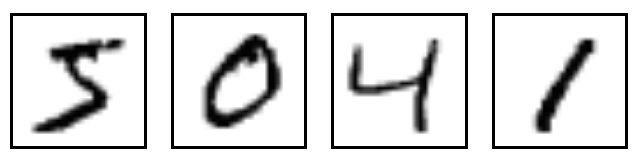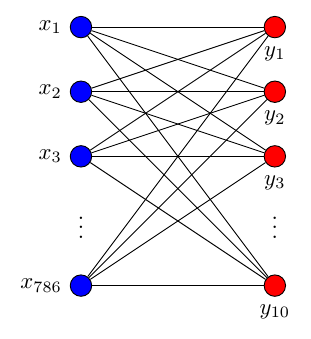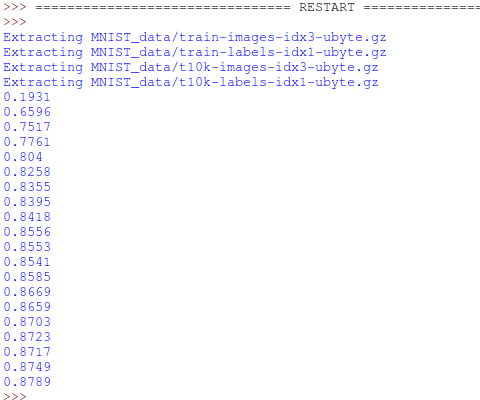# Classification 分类学习

## MNIST 数据 ¶

from tensorflow.examples.tutorials.mnist import input_data


MNIST库是手写体数字库，差不多是这样子的## 搭建网络 ¶

xs = tf.placeholder(tf.float32, [None, 784]) # 28x28


ys = tf.placeholder(tf.float32, [None, 10])


prediction = add_layer(xs, 784, 10, activation_function=tf.nn.softmax)## Cross entropy loss ¶

loss函数（即最优化目标函数）选用交叉熵函数。交叉熵用来衡量预测值和真实值的相似程度，如果完全相同，它们的交叉熵等于零。

cross_entropy = tf.reduce_mean(-tf.reduce_sum(ys * tf.log(prediction),
reduction_indices=)) # loss


train方法（最优化算法）采用梯度下降法。

train_step = tf.train.GradientDescentOptimizer(0.5).minimize(cross_entropy)
sess = tf.Session()
# tf.initialize_all_variables() 这种写法马上就要被废弃
# 替换成下面的写法:
sess.run(tf.global_variables_initializer())


## 训练 ¶

batch_xs, batch_ys = mnist.train.next_batch(100)
sess.run(train_step, feed_dict={xs: batch_xs, ys: batch_ys})


if i % 50 == 0:
print(compute_accuracy(
mnist.test.images, mnist.test.labels))